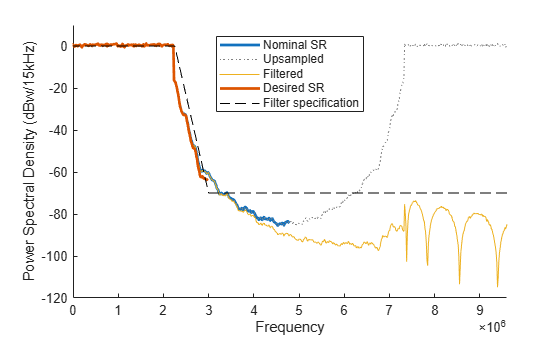This example describes the internal resampling filter design of the OFDM functions nrOFDMModulate, nrOFDMInfo, and nrOFDMDemodulate. These functions enable you to specify the OFDM sample rate and FFT size by using the SampleRate and Nfft name-value input arguments. When the specified sample rate and the nominal sample rate, corresponding to the FFT size, do not match, the OFDM functions resample the waveform using an internal multirate FIR filter.

OFDM Sample Rate and Nominal Sample Rate

The value that you set for the SampleRate input determines the sample rate of the waveform.

The nominal sample rate corresponding to the FFT size used in the OFDM modulation, ${\mathit{FFT}}_{\mathit{SR}}$, is equal to Nfft * carrier.SubcarrierSpacing * 1000, where carrier is the input argument of the function call, specifying the carrier configuration.

When the specified sample rate and the nominal sample rate do not match, the OFDM functions resample the waveform using an internal resampling filter. Setting the sample rate to the nominal sample rate does not result in resampling during OFDM modulation.

OFDM Modulation without Resampling

Create a carrier configuration object with 25 resource blocks.

carrier = nrCarrierConfig;
carrier.NSizeGrid = 25;

Get OFDM information.

ofdmInfo = nrOFDMInfo(carrier);

Display nominal sample rate and the sample rate returned in the OFDM information.

ofdmInfo.Nfft * carrier.SubcarrierSpacing * 1000
ans = 7680000
ofdmInfo.SampleRate
ans = 7680000

Verify that specifying a sample rate of 7.68e6 does not result in resampling during OFDM modulation.

grid = nrResourceGrid(carrier);
grid(:) = nrSymbolModulate(randi([0 1],numel(grid)*2,1),'QPSK');
waveform1 = nrOFDMModulate(carrier,grid);
waveform2 = nrOFDMModulate(carrier,grid,'SampleRate',7.68e6);
isequal(waveform1,waveform2)
ans = logical
1

Filter Design

The resampling filter is a function of these two ratios.

• The ratio of the transmission bandwidth (${\mathit{TX}}_{\mathit{BW}}$) to the nominal sample rate: ${\mathit{TX}}_{\mathit{BW}}$ / ${\mathit{FFT}}_{\mathit{SR}}$, where ${\mathit{TX}}_{\mathit{BW}}$ is equal to carrier.NSizeGrid * 12 * carrier.SubcarrierSpacing * 1000.

• The ratio of the nominal sample rate to the specified sample rate: ${\mathit{FFT}}_{\mathit{SR}}$ / SampleRate.

The OFDM functions call the designMultirateFIR function with these input arguments to design the resampling filter.

• Interpolation factor, L, equal to SampleRate/g, where g = gcd(${\mathit{FFT}}_{\mathit{SR}}$,SampleRate). Because the resampling of the OFDM-modulated waveform is by a factor of SampleRate/${\mathit{FFT}}_{\mathit{SR}}$, the resampling is costly if SampleRate and ${\mathit{FFT}}_{\mathit{SR}}$ do not have large common factors.

• Decimation factor, M, equal to ${\mathit{FFT}}_{\mathit{SR}}$/g.

• Transition width, TW, such that the transition band starts at $±$${\mathit{TX}}_{\mathit{BW}}$ / 2 (that is, the transition band starts at the edge of the occupied bandwidth).

• Stopband attenuation, Astop, equal to 70 dB.

If SampleRate > ${\mathit{FFT}}_{\mathit{SR}}$, then:

• L > M and the transition band stops at $±$${\mathit{FFT}}_{\mathit{SR}}$ / 2 (corresponding to a normalized frequency of 1/L).

• The filter acts as an anti-imaging filter after upsampling by L.

If SampleRate < ${\mathit{FFT}}_{\mathit{SR}}$, then:

• M > L and the transition band stops at $±$SampleRate/2 (corresponding to a normalized frequency of 1/M).

• The filter acts as an anti-aliasing filter before downsampling by M.

OFDM Modulation with Resampling

Create a carrier configuration object with 25 resource blocks.

carrier = nrCarrierConfig;
carrier.NSizeGrid = 25;

Compute the transmission bandwidth.

txBW = carrier.NSizeGrid * 12 * carrier.SubcarrierSpacing * 1000
txBW = 4500000

Specify a custom sample rate. Obtain the corresponding OFDM information and calculate the nominal sample rate for the default FFT size.

SampleRate = 6e6;
ofdmInfo = nrOFDMInfo(carrier,'SampleRate',SampleRate);
Nfft = ofdmInfo.Nfft
Nfft = 640
fftSR = Nfft * carrier.SubcarrierSpacing * 1000
fftSR = 9600000

Calculate g, L, and M.

g = gcd(fftSR,SampleRate)
g = 1200000
L = SampleRate / g
L = 5
M = fftSR / g
M = 8

Plot the spectrum of the OFDM waveform before resampling by setting the sample rate to the nominal sample rate.

figure;
hold on;
[f,S] = measureSpectrum(carrier,Nfft,fftSR,1);
plot(f,S,'LineWidth',2);

Plot the spectrum of the OFDM waveform after upsampling by L, before applying the filter.

[fL,S] = measureSpectrum(carrier,Nfft,fftSR,L);
hL = plot(fL,S,':');

Plot the spectrum of the OFDM waveform after applying the filter.

[f,S] = measureSpectrum(carrier,Nfft,fftSR*L,1);
plot(f,S);

Plot the spectrum of the final OFDM waveform after downsampling.

[f,S] = measureSpectrum(carrier,Nfft,SampleRate,1);
plot(f,S,'Color',hL.Color,'LineWidth',2);
hL.Color = [0.5 0.5 0.5];

Overlay the filter design specification.

fStop = fftSR / 2 * L / max([L M]);
aStop = -70.0;
spec = [fL(1) aStop; -fStop aStop; -txBW/2 0; txBW/2 0; fStop aStop; fL(end) aStop];
plot(spec(:,1),spec(:,2),'k--');
legend('Nominal SR','Upsampled','Filtered','Desired SR','Filter specification','Location','north');
xlabel('Frequency');
ylabel(['Power Spectral Density (dBw/' num2str(carrier.SubcarrierSpacing) 'kHz)']);
axis([0 fftSR -120 10]);Local Function

function [f,S] = measureSpectrum(carrier,Nfft,SampleRate,L)

% Subcarrier spacing in Hz
SCS = carrier.SubcarrierSpacing * 1e3;

% Set up spectrum estimator
spectrumEstimator = dsp.SpectrumEstimator;
spectrumEstimator.SampleRate = SampleRate;
spectrumEstimator.AveragingMethod = 'Exponential';
spectrumEstimator.ForgettingFactor = 0.99;
spectrumEstimator.FrequencyRange = 'centered';
spectrumEstimator.PowerUnits = 'dBW';
spectrumEstimator.SpectrumType = 'Power density';
spectrumEstimator.FFTLengthSource = 'Property';
spectrumEstimator.FFTLength = floor(SampleRate * L / SCS);

% For 100 slots
rs = RandStream('mt19937ar','Seed',1);
for nSlot = 0:99

% Create a slot grid filled with QPSK symbols and OFDM modulate
grid = nrResourceGrid(carrier);
grid(:) = nrSymbolModulate(rs.randi([0 1],numel(grid)*2,1),'QPSK');
waveform = nrOFDMModulate(carrier,grid,'Nfft',Nfft,'SampleRate',SampleRate);
waveform = waveform * Nfft;

% Apply interpolation if required
if (L>1)
T = size(waveform,1);
waveform = reshape([waveform.'; zeros(L-1,T)],[],1) * sqrt(L);
end

% Measure the spectrum
S = spectrumEstimator(waveform);

end

% Create frequency axis
N = spectrumEstimator.FFTLength;
f = (-N/2:(N/2 - 1)) * SCS;

% Translate from dBw/Hz to dBW/SCS
S = S + 10*log10(SCS);

end